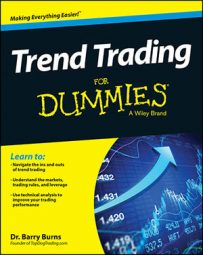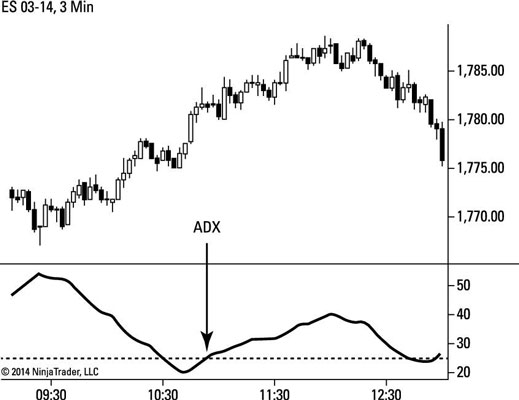ADX measures trend strength by calculating a moving average (typically the 14-period moving average) of price expansion, using average true range. These terms are defined in the following list:

• A moving average is the average of the closing prices of a set number of previous bars. For example, to calculate the average of the last 14 days of a market, you simply add the closing price of the last 14 days and then divide by 14.

To create a moving average, you calculate the average of every day and plot the series of those values on the chart. The result is a line on the chart as price continues to move into the future with each new day.

• A period, with reference to a moving average, is the time interval of each bar you’ve selected for that chart. A 14-period average on a daily chart (each bar representing one day of price action) is the average of the closing price of the last 14 days.

A 14-period average on a five-minute chart (each bar representing five minutes of price action) is the average of the closing price of the last 14 five-minute bars.

• Average true range measures price volatility over a period of time or stated number of bars (a typical default is 14 bars). Volatility measures the range of market moves from high to low but doesn’t directly reference the direction of those moves.

J. Welles Wilder suggests that the objective signal for a trend, using ADX, is when its value is above 25, but some traders prefer to use a value of 20. This signal doesn’t indicate whether the market is in an uptrend or a downtrend, simply that the market is in a strong trend. Thus, the indicator is popular for being simple, objective, and easy to interpret.

The figure shows the ADX line providing clear and objective readings for a strong trend (up or down) when it crosses above the value of 25.Credit: Figure by Barry Burns